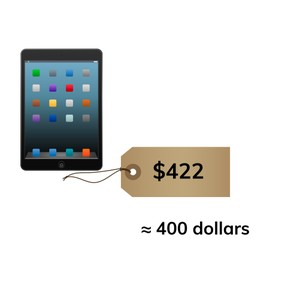Rounding to the nearest ten and hundred- numbers to 1,000

# Rounding to the nearest ten and hundred- numbers to 1,000

Rounding to the nearest ten and hundred- numbers to 1,0008,000 schools use Gynzy92,000 teachers use Gynzy1,600,000 students use Gynzy

## General

Students learn to round to the nearest ten and hundred of numbers to 1,000.

## Relevance

It is important to be able to round numbers, so you can calculate faster and make estimations. An example is if you want to buy a tablet that costs \$422, you know that you need about \$400.

## Introduction

Practice skip counting in 10s forwards and backwards to 100. Toss the ball to a student who says "10". They throw to the next student, who says "20". Continue to 100. Then restart at 100 and skip count in 10s back to 0.

## Development

The interactive whiteboards shows the tens and hundreds. Discuss these with your students and say they can be called landmark numbers. Explain that by rounding a number you look to see what the closest ten or hundred is.
Students use a number line to see which ten or hundred they round up or down to. They can do this by first looking for the nearest ten, and then to look for the nearest hundred. Students can see on the number line which ten or hundred is closest in proximity to the given number.
Next, explain to students that you round up when a number is 5 or greater and you round down if the number is 4 or fewer. By dragging the number into the HTO(hundreds, tens, ones) chart students can see the place values of a number. Discuss with students which number they need to look at to do their rounding. If they round to a ten, they have to look at the ones place. If they round to a hundred, they need to look at the tens place. Practice doing so as a class with two numbers and round them to both tens and hundreds.

To check that students understand rounding numbers to 1,000 to tens and hundreds, you can ask the following questions:
- What are tens?
- What are hundreds?
- Which number do you look at if you want to round to a ten?
- Which number do you look at if you want to round to a hundred?
- When do you round up or round down?

## Guided practice

Students first practice rounding up or down by answering multiple choice questions. They then must drag a number to its nearest hundred. Ask students to explain which digit they look at to determine if they round up or down.

## Closing

Discuss with students what the importance of rounding is, namely that you learn to estimate. Check their understanding using the final exercise on the interactive whiteboard of rounding to the nearest ten. Show numbers one by one. When the number shown should be rounded up, students stand up. When the number shown should be rounded down, students sit down. Repeat the fact that students should look at the ones if they are rounding to a ten, and at the tens if they are rounding to a hundred.

## Teaching tips

Students who have difficulty rounding can use the HTO chart and/or a number line.

### The online teaching platform for interactive whiteboards and displays in schools

• Save time building lessons

• Manage the classroom more efficiently

• Increase student engagement